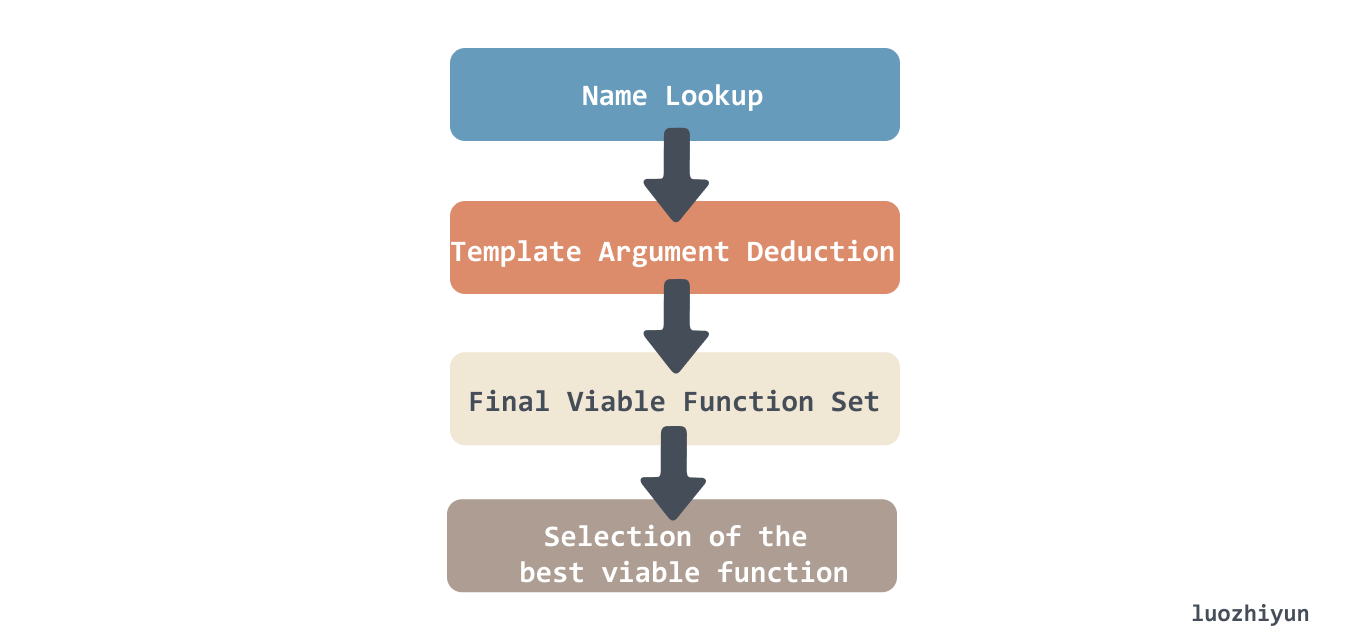# C++ 中复杂却很有意思的SFINAE技术

SFINAE 其实就是重载的函数模板匹配，编译器根据名称找出所有适用的函数和函数模板，然后要根据实际情况对模板形参进行替换，在编译过程中寻找一个最佳匹配的过程。

``````struct Test {
typedef int foo;
};

template <typename T>
//要求类型T定义了内嵌类型foo
void f(typename T::foo) {} // Definition #1

template <typename T>
void f(T) {}               // Definition #2

int main() {
f<Test>(10); // Call #1.
f<int>(10);  // Call #2. Without error (even though there is no int::foo) thanks to SFINAE.
return 0;
}``````

• 首先是根据执行名称进行查找；
• 对于函数模板来说，模板参数是根据传入的参数类型来进行推断的；
• 根据传入类型找到对应模板之后会执行参数类型替换，并加入到解析集中；
• 如果找到对应的类型不符合则从解析集中删除；
• 在最后，我们可以得到这个函数调用的解析集；
• 如果解析集是空的，那么就编译失败，比如我们把上面例子中的`Definition #2`模板删除了，那 `f<int>(10);`调用就会编译失败；
• 如果解析集有一个以上，那么需要根据参数类型找到最合适能被匹配上的函数；``````template <typename T>
struct has_reserve {
struct good { char dummy; };
struct bad { char dummy; };

template <class U, float (U::*)()>
struct SFINAE {};

template <typename  U>
static good test(SFINAE<U, &U::reserve>*);

template <typename>

static const bool value = sizeof(test<T>(nullptr)) == sizeof(good);
};

class  TestReserve {
public:
float reserve();
};

class Bar {
public:
int type;
};

int main() {
cout << "reserve:" << has_reserve<TestReserve>::value << endl;//reserve: 1
cout << "reserve:" << has_reserve<Bar>::value << endl; //reserve:  0
return 0;
} ``````

## enable_if

``````// 1. the return type (bool) is only valid if T is an integral type:
template <typename T>
typename std::enable_if<has_reserve<T>::value,void>::type
reserve_test1 () {cout << "reserve_test1"<< endl;}

// 2. the second template argument is only valid if T is an integral type:
template < typename T,
typename = typename std::enable_if<has_reserve<T>::value>::type>
void reserve_test2 () {cout <<"reserve_test2" << endl;}

int main() {
reserve_test1<TestReserve>();
reserve_test2<TestReserve>();
return 0;
} ``````

1. 返回值类型使用`enable_if`
2. 模板参数额外指定一个默认的参数`class = typename std::enable_if<…>::type`

``error: no type named ‘type’ in ‘struct std::enable_if<false, void>’``

``````template <typename T>
typename std::enable_if<!has_reserve<T>::value,void>::type
reserve_test1 ()  {cout << "is not reserve " << endl;}

int main() {
reserve_test1<Bar>();
return 0;
} ``````

``````template <bool, typename T=void>
struct enable_if {
};

template <typename T>
struct enable_if<true, T> {
using type = T;
};``````

``````template <bool _Test, class _Ty = void>
using enable_if_t = typename enable_if<_Test, _Ty>::type;``````

`enable_if_t`就是`enable_if::type`的重定义，如果enable_if_t<_Test,_Ty>的 Test 为 true，可以看出走了 enble_if 的特化版本，有 type 的定义，否则就没有 type 这个定义，有了这个版本之后实际上我们就可以把上面例子中的 `::type`去掉，稍微简化一下代码，这个也是可以在很多地方看到有用的。

## decltype & std::declval

decltype 是“declare type”的缩写，译为声明类型 ，decltype 可以认为与 auto 关键字一样，用于进行编译时类型推导，但是 decltype 的类型推导并不是像 auto 一样是从变量声明的初始化表达式获得变量的类型，而是总是以一个普通表达式作为参数，返回该表达式的类型,而且decltype并不会对表达式进行求值。简单的用法如下：

``````int i = 4;
decltype(i) a; //推导结果为int。a的类型为int。``````

decltype 还有一个返回类型后置语法，将 decltype 和 auto 结合起来完成返回值类型的推导：

``````template <typename T, typename U>
auto add(T t, U u) -> decltype(t + u){
return t + u;
}``````

``````struct A {
A() = delete;
int foo();
};

int main() {
decltype(A().foo()) foo = 1; // 无法通过编译
}``````

``````#include <utility>

struct A {
A() = delete;
int foo();
};

int main() {
decltype(std::declval<A>().foo()) foo = 1; // OK
}``````

``````template <typename  U>
auto test() ->decltype(declval<U&>().reserve(),void())
{
cout << "type " << endl;
}

int main() {
test<TestReserve>();
return 0;
} ``````

declval 可以在某类型没有默认构造函数的情况下，假想出一个该类的对象来进行类型推导。所以 `declval<U&>().reserve()` 用来测试 `U&` 类型的对象是不是有 reserve 成员函数。

## void_t

void_t 的定义如下：

``````template <typename...>
using void_t = void;``````

``````template< class , class = void >
struct new_has_reserve : std::false_type
{ };

template< class T >
struct new_has_reserve< T , void_t< decltype(declval<T&>().reserve() ) > > : std::true_type
{ };``````

``````class A {
public:
int reserve();
};

class B {
};

int main() {
cout << new_has_reserve< A >::value << endl; // 1
cout <<new_has_reserve<B>::value << endl; // 0
return 0;
} ``````

``````template< class , class = void >
struct new_has_reserve : std::false_type
{ };``````

``````template< class T >
struct new_has_reserve< T , void_t< decltype(declval<T&>().reserve() ) > > : std::true_type
{ };``````

`new_has_reserve< A >::value` 去匹配的时候，对于第一个模板参数来说 T 是可以被推导为 A 的；

``````template< class T >
struct new_has_reserve< A , void ) > > : std::true_type
{ };``````

## constexpr

constexpr 它是在 C++ 11 被引进的，它的字面意思是 constant expression，常量表达式。它可以作用在变量和函数上。一个 constexpr 变量是一个编译时完全确定的常数。一个 constexpr 函数至少对于某一组实参可以在编译期间产生一个编译期常数。

``````template<int N> class C{};

constexpr int FivePlus(int x) {
return 5 + x;
}

void f(const int x) {
C<x> c1;            // Error: x is not compile-time evaluable.
C<FivePlus(6)> c2;  // OK
} ``````

### C++17 & if constexpr

``````template <class T> constexpr T absolute(T arg) {
return arg < 0 ? -arg : arg;
}

template <class T>
constexpr enable_if_t<is_floating_point<T>::value, bool>
close_enough(T a, T b) {
return absolute(a - b) < static_cast<T>(0.000001);
}
template <class T>
constexpr enable_if_t<!is_floating_point<T>::value, bool>
close_enough(T a, T b) {
return a == b;
}``````

``````template <class T> constexpr T absolute(T arg) {
return arg < 0 ? -arg : arg;
}

template <class T>
constexpr auto precision_threshold = T(0.000001);

template <class T> constexpr bool close_enough(T a, T b) {
if constexpr (is_floating_point_v<T>)
return absolute(a - b) < precision_threshold<T>;
else
return a == b;
}``````

## Reference

https://izualzhy.cn/SFINAE-and-enable_if

https://zhuanlan.zhihu.com/p/21314708

https://time.geekbang.org/column/intro/100040501

https://stackoverflow.com/questions/9939305/what-is-in-c

https://www.zhihu.com/question/51441745

https://stdrc.cc/post/2020/09/12/std-declval/

https://offensive77.plus/index.php/2021/12/04/history-von-sfinae/

https://www.cppstories.com/2016/02/notes-on-c-sfinae/

https://www.cppstories.com/2018/03/ifconstexpr/

https://stackoverflow.com/questions/27687389/how-does-void-t-work

https://akrzemi1.wordpress.com/2013/06/20/constexpr-function-is-not-const/

https://www.zhihu.com/question/35614219